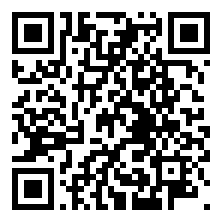Code Review: StringDataLeoZ 7月 25, 2019
• 在其它设备中阅读本文章
•## Reviews

### Classic methods:

• S+S link string itself [Rotated Word]
• Sort or count char counts to determine two strings

### Grammer tips:

1. split() will split by space(not just one space)
2. strip() can cut space at head&end
3. reversed() will have return, reverse() just reverse itself but no return.
4. islower(), upper()
5. ASCII ord(‘a’) -32 = ‘A’
6. ASCII array(127)
7. A.difference(B)

## Problems

1. 8. Rotate String [Solution]
Rotate the char array in place by offset.
The rest ‘offset’ numbers of char will be move to head. So classic method, link two same string and get result start from proper index.
Make sure consider the offset more than len(array) first.
Space O(n), Time O(n)

2. 13. Implement strStr() [Solution]
Find target string in string.
Python has target() function, while the normal way to implement is is O($n^2$) time to find it. KMP / Rabin Karp not considered yet.

3. 53. Reverse Words in a String [Solution]
Reverse words in sentence.
4. 133. Longest Word [Solution]
record a tmp max_length, loop all words, if len(word) > max, update max_length, and empty max_words array, insert this ‘new max’ word.
5. 146. Lowercase to Uppercase II [Solution]
char convert
6. 157. Unique Characters [Solution]
with extra data structure, use set to comapre. Or build a ASCII array(127 all, but use 129 instead). Loop check wether the code has been used.
7. 158. Valid Anagram [Solution]
anagrams problem. Stright method, sort two strings, see weather they are equal or not. But require 2 O(LogN) time at least. If require O(n) time, O(1) extra space. Just caculate each char counts in two strings, if occur diff, return false.
8. 200. Longest Palindromic Substring [Solution]
O(n2), DP lopp fiind from possible longest string is a way. 另有中心线枚举和Manacher’s Algorithm in O(N) time.
9. 209. First Unique Character in a String [Solution]
hash map record each char counts, loop char in string return the fist char occur 1 time only. [?Considering Data Stream problem?]
10. 211. String Permutation [Solution]
Sort or count char counts to determine two strings
11. 212. Space Replacement [Solution]
Reverse Loop.
12. 241. String to Integer [Solution]
start from last index to add. or use ord to determine number in char.
13. 408. Add Binary [Solution]
[Star problem in string]reverse calc sum, not hard to come up method, but need to pay attension details.
14. 415. Valid Palindrome [Solution]
normal way, build a clean string, jungle weather it is a paindrome string, need extra space. O(n) time without extra memory. Need use two pointers method.
15. 420. Count and Say [Solution]
print a string as how to say it.
recursion cout and print rule string.
16. 422. Length of Last Word [Solution]
return the length of last word.
17. 425. Letter Combinations of a Phone Number [Solution]
DFS recursion find all possible combination.
18. 449. Char to Integer [Solution]
ord() return char’s ASCII
19. 478. Simple Calculator [Solution]
Do as calculator.
20. 491. Palindrome Number [Solution]
[Star problem in string]Negative is not palindrome. Some hints:
Could negative integers be palindromes? (ie, -1)
If you are thinking of converting the integer to string, note the restriction of using extra space.
You could also try reversing an integer. However, if you have solved the problem “Reverse Integer”, you know that the reversed integer might overflow. How would you handle such case?
There is a more generic way of solving this problem.
21. 524. Left Pad [Solution]
build string as required.
22. 671. Rotate Words [Solution] [Review]
Check rotated words. Note that use 2 string method to check rotated words will only in 2len(word) time.
23. 702. Concatenated String with Uncommon Characters of Two Strings [Solution] [Review]
Concatenate uncommon char in two strings.[Official answer is better]
24. 720. Rearrange a String With Integers [Solution]
seperate deal nums and upper char, then merge
25. 891. Valid Palindrome II [Solution]
judge palindrome if allows to delete at most one cahr. Two pointers to jundge string, if occur not equal char, jundge delete left or right pointer’s char, see weather it can be palindrome.
26. 914. Flip Game [Solution]
enumerate all possible ++ postion, build string and add them in results.
27. 1011. Number of Lines To Write String [Solution] store chars in multi lines area. Note if line will exceed 100 units after inout current char, just move it to store in next line.
28. 1013. Unique Morse Code Words [Solution]
Convert Each words to morser code, add in set() to see unique counts.
29. 1079. Count Binary Substrings [Solution]
continue binary search. Key point is if find the mid point(01, 10) then continue count when ‘i-start<lastcount’. Pay attention to border situation.
30. 1104. Judge Route Circle [Solution]
Just follow the rule
31. 1173. Reverse Words in a String III [Solution]
Basic string operation
32. 1193. Detect Capital [Solution]
Consider all possible situation.
33. 1204. Keyboard Row [Solution]
A.difference(B) can figure this problem
34. 1243. Number of Segments in a String [Solution]
遍历整个字符串
一个字符串段落的特征是：
当前位置的字符不为’ ‘并且(前一个字符为’ ‘或者当前位置是第0位）
35. 1266. Find the Difference [Solution]
ASCII ord() will be a interesting solution.
36. 1283. Reverse String [Solution]
Basic string operation
37. 1350. Excel Sheet Column Title [Solution]
[Star problem in string] like 26 Decimal system.
38. 1638. Least Substring [Solution]
这道题字符串分段有两种情况
当前相同字符长度为k，或者字符不匹配
用for循环更新flag字符和新段子的字符长度
39. 1713. Unique Email Addresses [Solution]
Basic string operation

This blog is under a CC BY-NC-SA 3.0 Unported License